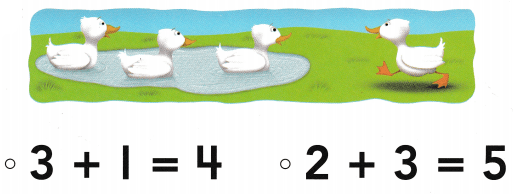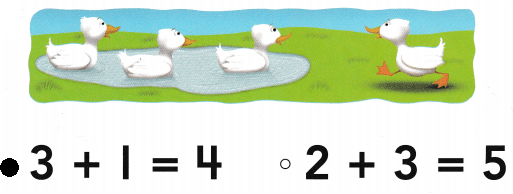# Texas Go Math Kindergarten Lesson 11.4 Answer Key Addition Word Problems

Refer to our Texas Go Math Kindergarten Answer Key Pdf to score good marks in the exams. Test yourself by practicing the problems from Texas Go Math Kindergarten Lesson 11.4 Answer Key Addition Word Problems.

## Texas Go Math Kindergarten Lesson 11.4 Answer Key Addition Word Problems

Unlock the Problem

DIRECTIONS: Listen and use counters to act out the addition word problem. Write the addition sentence. Tell a friend how many children in all.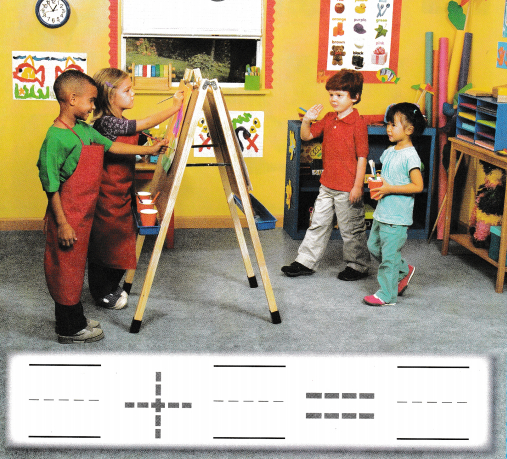Explanation:
2 children  are drawing 2 more child joined them
2 + 2 = 4
so, there are 4 children in all.

Try Another Problem

DIRECTIONS: 1. Listen to and use counters to act out the addition word problem. Write the addition sentence.

Question 1.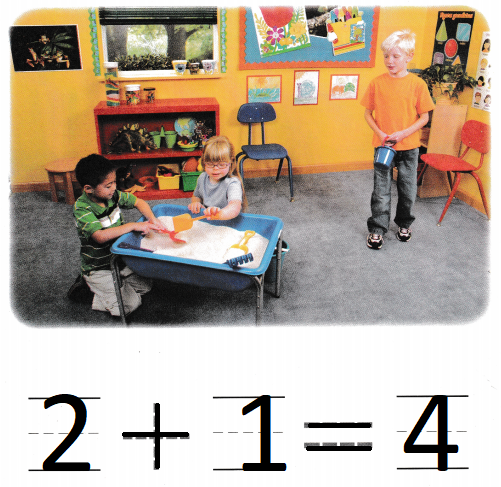Explanation:
2 children are playing sand art and one more child joined them
2 + 1 = 4
so, 3 children are there.

Share and Show

DIRECTIONS: 2. Listen to and use counters to act out the addition word problem. Write the numbers and trace the symbols to complete the addition sentence.

Question 2.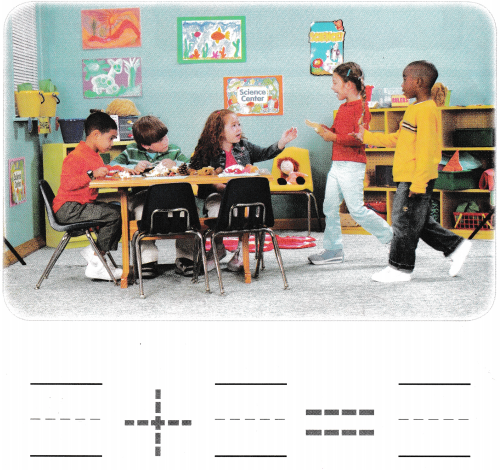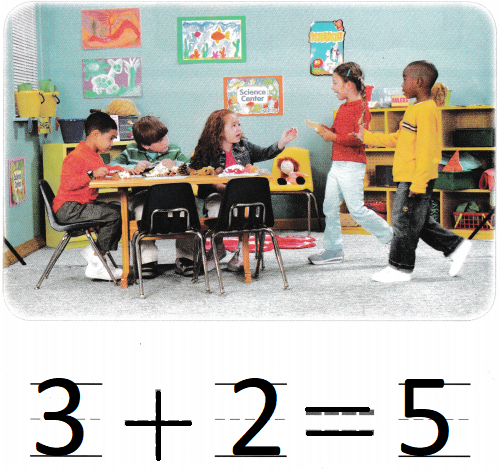Explanation:
3 children are drawing
and 2 more children joined them
3 + 2 = 5
so, there are 5 children in all.

HOME ACTIVITY • Tell your child a short word problem about adding three objects to a set of two objects. Have your child use toys to act out the word problem.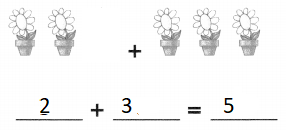Explanation:
There are 2 flower pots
and 3 more flower pots
2 + 3 = 5
so, there are 5 flower pots in all.

DIRECTIONS: Choose the correct answer. 3. A fish is swimming. Another fish swims behind it. Act out 3 + 1. How many fish are there? 4. Three birds are on a branch. Another bird joins them. Act out 3 + 1. How many birds are there? 5. Two butterflies are on a flower. Two more butterflies come. Act out the problem. What does the picture show?

Question 3.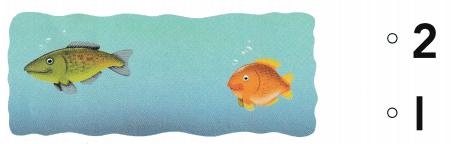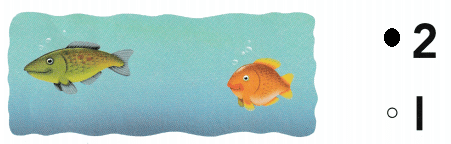Explanation:
A fish is swimming.
1+ 1 = 2
so, there are 2 fishes swimming

Question 4.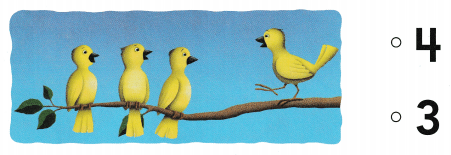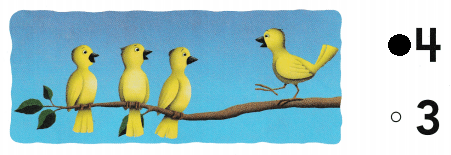Explanation:
Three birds are on a branch.
Another bird joins them
3 + 1 = 4
so, there are 4 birds in all.

Question 5.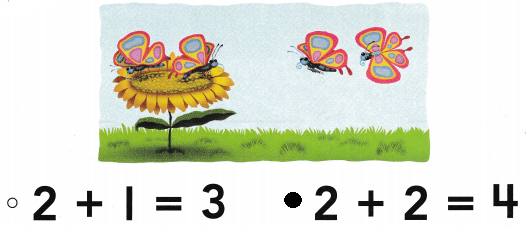Explanation:
Two butterflies are on a flower.
Two more butterflies come
2 + 2 = 4
so, there are 4 butter flies in all.

### Texas Go Math Kindergarten Lesson 11.4 Homework and Practice Answer Key

DIRECTIONS: 1. One fish ¡s swimming. Two more fish join. Draw to show the addition word problem. Write the numbers and trace the symbols to complete the addition sentence.

Question 1.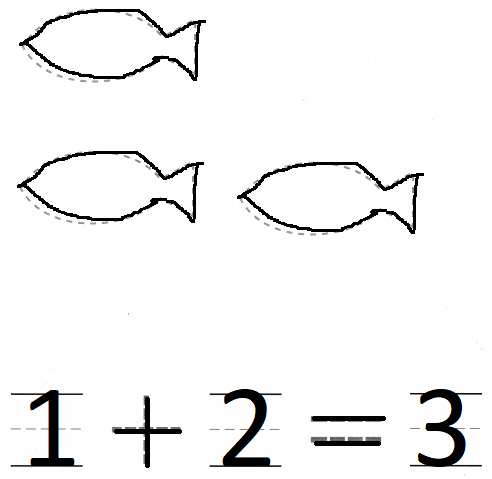Explanation:
One fish ¡s swimming. Two more fish join
1 + 2 = 3
so, there are 3 fishes swimming

DIRECTIONS: Choose the correct answer. 2. Two puppies are eating. Another puppy joins them. How many puppies are there? 3. Three ladybugs are on a leaf. Two more ladybugs join them. How many ladybugs are there? 4. Three ducks are in a pond. One more duck comes. What does the picture show?

Lesson Check

Question 2.Explanation:
Two puppies are eating.
Another puppy joins them.
There are 3 puppies in total.

Question 3.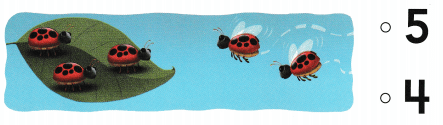Explanation:
Three ladybugs are on a leaf.
3 + 2 = 5
so, 5 bugs on the leaf.

Question 4.